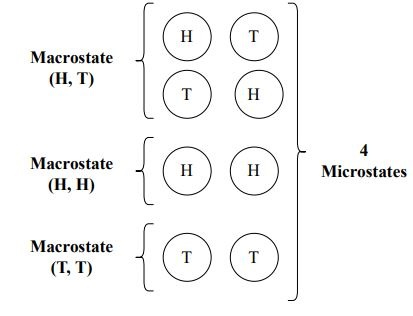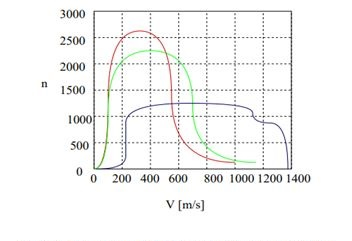# Statistical Physics

## Introduction

Statistical physics is defined as the physics plot which has taught humans with multiple practical modules. Statistical physics utilises the procedures of statistics and probability theory. The mathematical tools especially help for managing large populations and mitigating the problems of physics. Statistical physics help in illustrating the wide fields with the inherent stochastic nature. The main purpose of statistical physics is to clarify the matter properties concerning physical laws governing the motion of atoms. Statistical physics illustrates and quantitatively discusses super fluidity, turbulence, superconductivity, a collective condition in plasma and solids and the structural characteristics of a liquid.

## What is Statistical Physics?

Statistical physics has the aim of acknowledging the system's macroscopic factors in the form of equilibrium. It is demonstrated from the information on the properties of microscopes through the mechanic's law (Kolchinsky & Wolpert, 2018). The process is not similar to the states of thermodynamics that diagnose the system of a microscope in equilibrium state from the position of the microscope except for seeing the parameters of a microscope.

Images Coming soon

The statistical division is defined as the division where the system is placed in determining free energy. Statistical physics manages the motion of atoms and surpluses of physics law based on the data of microscopic laws (Gorban & Tyukin, 2018). Statistical physics assists in learning both the states of thermal equilibrium and nonequilibrium. The application of statistics helps to illustrate random procedures like thermodynamics like Brownian motion.

## What is the Necessity of Statistical Description?

Statistical descriptions are defined as the brief descriptive coefficient that concludes a provided set of data which can be a population sample or an entire population's representation. As opined by Hou & Huang (2020), descriptive statistics are broken down into the computing of variability and central tendency. Considering the instance of studying glass, a statistical distribution of velocities of particles is examined and gained in comprehending the connection between the macroscopically visible qualities like temperature, volume and pressure.The statistical description provides an important insight into better comprehension and exact description. The physics branch is where a particular scheme to measure free energy is measured (Kolchinsky & Wolpert, 2018). In understanding statistical physics, statistical mechanics amalgamates the process and principles of statistics concerning the thermodynamics field. In physics, mainly statistical descriptions are highly focused to make the study of particles more elaborated than the descriptive study.

## Analysis of qQuantum and Mathematical-Statistical Physics

Quantum statistical physics or mechanics of quantum statistics in modern physics is referred to as the statistical mechanics beneficial in the systems of quantum mechanics. The statistical ensemble or probability circulation over the potential states of quantum in quantum mechanics is demonstrated by the operator of density which is self-adjoin, trace class machinist and non-negative of trace 1 on the space of Hilbert narrating the system of quantum. It is demonstrated that no concepts beyond statistics are required for quantum physics (Azha et al. 2018). The distinctions in this are only apparent and consequences of the specialities of the systems of classical statistics help in acknowledging the descriptions of quantum mechanics. Quantum statistics are the statistics associated with the distinction of the identical elementary particles, atoms and ions' numbers among the probable states of quantum.

Statistical physics in mathematics is represented as the mechanics of statistics. It was presented by J Willard Gibbs in 1902. The group of statistics is mentioned as the magnification that comprises a huge amount of the system's virtual copies and all calculated simultaneously. Everything in the group of statistics signifies a possible state that real system nights have occurred. Basic probability theory focuses on the mathematical parts of statistical physics (Luginbuhl, Rundle & Turcotte, 2019). Some of the concepts regarding probability distributions, permutations, variance and mean depend on mathematical-statistical physics.

## Application of Statistical PhysicsStatistical physics has helped in gaining a proper insight into broad processes range like diffusion. As cited by Ray (2019), the application of the statistical approach to thermodynamics may lead to in-depth comprehension of concepts such as entropy and temperature. For instance, the temperature is comprehended statistically as the average atoms' kinetic energy in a bulk material. Applications of statistical physics are demonstrated below:

• The application of the principal statistical physics is centred on the molecules' dissemination in collecting. Statistical physics is highly functional in the distribution of Maxwell of molecular velocity.

• The particle density is low if the temperature is high.

• Gibbs has highlighted the facts of thermodynamics and its other aspects in the context of statistical physics.

## Conclusion

Statistical physics in solid-state physics supports the study of crucial conditions, phase transformation and liquid crystals. Statistics are more feasible and mechanics are mostly preferred in the study of engineering or physics. Statistical physics can be highly complementary in both subjects. Statistical physics is relatively easier, nevertheless, physics needs quick utilisation of IQ in comparison to physics. In physics, statistical description assists in structuring the efficient and proper planning of the statistical inquiry in a distinct field. Statistical physics has proved to be beneficial in the derivation of the formulation of the path integral of quantum physics.

## FAQs

Q.1. What is the primary postulation of Statistical mechanics?

Ans. The suggestion is generally known as the equality principle which is based on probability theory. It commanded that particles and volumes’ numbers can emerge with similar frequency in the chorus if the microstates have equal energy.

Q.2. What is the major application of Statistical physics?

Ans. Statistical physics' formalism enables an application of the general consequences to different branches such as economy, biology and solid-state theory. The application of statistical physics in practical life is quite interesting.

Q.3. What are the types of statistical physics?

Ans. Statistical mechanics of ideal gas, entropy, quantum statistics, application to radiation and many more are the basic concepts of statistical physics. Statistics provides a high degree of freedom from the formative concepts.

Q.4. What is the distinction between thermodynamics and statistical mechanics?

Ans. Statistical mechanics or physics is referred to as the concept of physical performance of a macroscopic initiating from the data of the forces of a microscope amongst the fundamental particles. On the other hand, thermodynamics is referred to as the connection concept between different macroscopic visibility such as pressure, temperature, polarisation and magnetisation of the system.